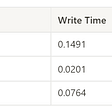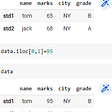# Different ways to iterate a dataframe in pandas

Many a time we need to iterate our dataframe. Mostly when we need to change any particular cell value or need to check some conditional statement. Actually there’re hundreds of reason you may need to check cell by cell values in pandas dataframe. There’s several ways to do this. The number of ways I tried will show here. And obviously show the best and easy way according to me.

1. Using loc: This is location method of pandas dataframe. Here you need to tell the location and index of the cell. This is one of the easiest way. Let’s see how can we do it.

import pandas as pd
data = {‘Name’: [‘Polok’, ‘Shaiful’, ‘Maruf’, ‘Naim’],
‘IsAlumni’: [‘Yes’, ‘No’, ‘Yes’, ‘Yes’],
‘Contact’: [911, 206, 121, 666]}

# Convert the dictionary into dataframe
df = pd.DataFrame(data, columns = [‘Name’, ‘IsAlumni’, ‘Contact’])
for i in range(len(df)) :
print(df.loc[i, “Name”], df.loc[i, “IsAlumni”])

2. Using iloc: pandas dataframe supports this integer location system. Here it takes both row’s and column’s integer index value to iterate the cells of the dataframe. Let’s have a look.

import pandas as pd
data = {‘Name’: [‘Polok’, ‘Shaiful’, ‘Maruf’, ‘Naim’],
‘IsAlumni’: [‘Yes’, ‘No’, ‘Yes’, ‘Yes’],
‘Contact’: [911, 206, 121, 666]}

# Convert the dictionary into dataframe
df = pd.DataFrame(data, columns = [‘Name’, ‘IsAlumni’, ‘Contact’])
for i in range(len(df)) :
print(df.iloc[i, 0], df.iloc[i, 2])

3. Using iterrows: In this method it iterates every row of the dataframe and then iterates the rows by index value to access the determined cell. Below is the example.

import pandas as pd
data = {‘Name’: [‘Polok’, ‘Shaiful’, ‘Maruf’, ‘Naim’],
‘IsAlumni’: [‘Yes’, ‘No’, ‘Yes’, ‘Yes’],
‘Contact’: [911, 206, 121, 666]}

# Convert the dictionary into dataframe
df = pd.DataFrame(data, columns = [‘Name’, ‘IsAlumni’, ‘Contact’])
for index, row in df.iterrows():
print (row[“Name”], row[“IsAlumni”])

4. Using itertuples: In this method it iterates every row of the dataframe same as iterrows() but the difference is it doesn’t need to call the index in the for loop. Instead it uses a function getattr() where you need to pass iterator name and column name. See the example to get understand.

import pandas as pd
data = {‘Name’: [‘Polok’, ‘Shaiful’, ‘Maruf’, ‘Naim’],
‘IsAlumni’: [‘Yes’, ‘No’, ‘Yes’, ‘Yes’],
‘Contact’: [911, 206, 121, 666]}

# Convert the dictionary into dataframe
df = pd.DataFrame(data, columns = [‘Name’, ‘IsAlumni’, ‘Contact’])
for row in df.itertuples():
print (getattr(row, “Name”), getattr(row, “IsAlumni”))

5. The Easiest and best way to me: Most easily you can do it using index attribute of dataframe. Using a simple iterator in for loop you can easily do it. The below code shall give you a better understanding.

import pandas as pd
data = {‘Name’: [‘Polok’, ‘Shaiful’, ‘Maruf’, ‘Naim’],
‘IsAlumni’: [‘Yes’, ‘No’, ‘Yes’, ‘Yes’],
‘Contact’: [911, 206, 121, 666]}

# Convert the dictionary into dataframe
df = pd.DataFrame(data, columns = [‘Name’, ‘IsAlumni’, ‘Contact’])
for ind in df.index:
print(df[‘Name’][ind], df[‘IsAlumni’][ind])

These are the methods I have used to iterate over a dataframe. The last one I use the most. Hope this would help you too.

--

--

--

## More from Md. Hasibul Islam

I’m a data science enthusiast. Always try to cope up with the upgraded technologies. Connect Me through polok.hasibul@gmail.com.

## Recommended from Medium## My First Medium Blog….## Communication Can Make or Break a Data Science Project## How data-driven innovation is fueling cities’ COVID response## How to Use Pivot Tables in Confluence## Do Hong Kongers still dine out under COVID-19?## Data Cleansing and Migration For Small Businesses## “Wildfire” Science-Research, September 2021, Week 4 — summary from Europe PMC## Md. Hasibul Islam

I’m a data science enthusiast. Always try to cope up with the upgraded technologies. Connect Me through polok.hasibul@gmail.com.

## Chapter 2: 11 questions on how to read/write files in Pandas. [Pandas Tutorials QnA Approach]